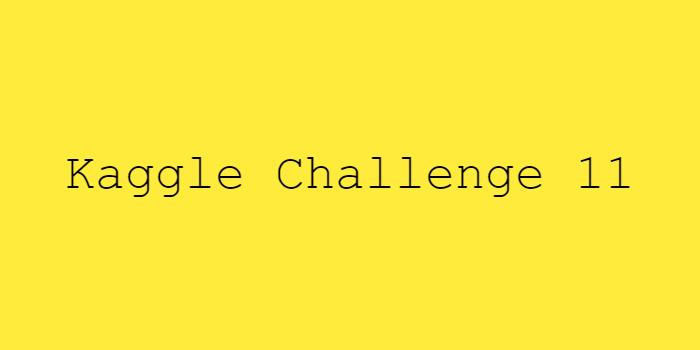# Kaggle Challenge 11 - Machine Learning Competitions

ljsk99499·2021년 8월 14일
0

## Kaggle Challenge August 2nd~

목록 보기
9/12# Kaggle Challenge 11 - Machine Learning Competitions

### 1. Housing Prices Competition

#### 1) Import

# Set up code checking
from learntools.core import binder
binder.bind(globals())
from learntools.machine_learning.ex7 import *

# Set up filepaths
import os
if not os.path.exists("../input/train.csv"):
os.symlink("../input/home-data-for-ml-course/test.csv", "../input/test.csv") 

#### 2) Pandas

# Import helpful libraries
import pandas as pd
from sklearn.ensemble import RandomForestRegressor
from sklearn.metrics import mean_absolute_error
from sklearn.model_selection import train_test_split

# Load the data, and separate the target
iowa_file_path = '../input/train.csv'
y = home_data.SalePrice

# Create X (After completing the exercise, you can return to modify this line!)
features = ['LotArea', 'YearBuilt', '1stFlrSF', '2ndFlrSF', 'FullBath', 'BedroomAbvGr', 'TotRmsAbvGrd']

# Select columns corresponding to features, and preview the data
X = home_data[features]

# Split into validation and training data
train_X, val_X, train_y, val_y = train_test_split(X, y, random_state=1)

# Define a random forest model
rf_model = RandomForestRegressor(random_state=1)
rf_model.fit(train_X, train_y)
rf_val_predictions = rf_model.predict(val_X)
rf_val_mae = mean_absolute_error(rf_val_predictions, val_y)

print("Validation MAE for Random Forest Model: {:,.0f}".format(rf_val_mae))

#### 3) Question

# path to file you will use for predictions
test_data_path = '../input/test.csv'

# read test data file using pandas
test_data = ____

# create test_X which comes from test_data but includes only the columns you used for prediction.
# The list of columns is stored in a variable called features
test_X = ____

# make predictions which we will submit.
test_preds = ____

#### 4) Solution

# In previous code cell
rf_model_on_full_data = RandomForestRegressor()
rf_model_on_full_data.fit(X, y)

# Then in last code cell
test_data_path = '../input/home-data-for-ml-course/test.csv'
output.to_csv('submission.csv', index=False)